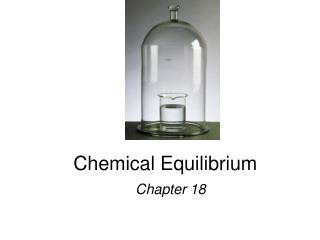DownloadDownload PresentationChemical Equilibrium

# Chemical Equilibrium

Download Presentation## Chemical Equilibrium

- - - - - - - - - - - - - - - - - - - - - - - - - - - E N D - - - - - - - - - - - - - - - - - - - - - - - - - - -
##### Presentation Transcript

1. Chemical Equilibrium Chapter 18

2. H2O (l) H2O (g) N2O4(g) 2NO2(g) Equilibrium is a state in which there are no observable changes as time goes by. • Chemical equilibrium is achieved when: • the rates of the forward and reverse reactions are equal and • the concentrations of the reactants and products remain constant Physical equilibrium Chemical equilibrium 15.1

4. constant 15.1

5. N2O4(g) 2NO2(g) K = aA + bB cC + dD [NO2]2 [C]c[D]d [N2O4] K = [A]a[B]b = 4.63 x 10-3 Law of Mass Action Equilibrium Will K >> 1 Lie to the right Favor products K << 1 Lie to the left Favor reactants 15.1

6. CH3COOH (aq) + H2O (l) CH3COO-(aq) + H3O+ (aq) ‘ ‘ Kc = Kc [H2O] General practice not to include units for the equilibrium constant. [CH3COO-][H3O+] [CH3COO-][H3O+] = [CH3COOH][H2O] [CH3COOH] Homogeneous Equilibrium [H2O] = constant Kc = 15.2

7. The equilibrium concentrations for the reaction between carbon monoxide and molecular chlorine to form COCl2 (g) at 740C are [CO] = 0.012 M, [Cl2] = 0.054 M, and [COCl2] = 0.14 M. Calculate the equilibrium constants Kc. CO (g) + Cl2(g) COCl2(g) = 0.14 0.012 x 0.054 [COCl2] [CO][Cl2] = 220 Kc= 15.2

8. CaCO3(s) CaO (s) + CO2(g) ‘ ‘ Kc = Kc x [CaO][CO2] [CaCO3] [CaCO3] [CaO] Heterogenous equilibrium applies to reactions in which reactants and products are in different phases. [CaCO3] = constant [CaO] = constant Kc= [CO2] = The concentration of solids and pure liquids are not included in the expression for the equilibrium constant. 15.2

9. CaCO3(s) CaO (s) + CO2(g) PCO PCO 2 2 does not depend on the amount of CaCO3 or CaO = Kp 15.2

10. N2(g) + 3H2(g) 2NH3(g) Equilibrium shifts left to offset stress Add NH3 Le Châtelier’s Principle If an external stress is applied to a system at equilibrium, the system adjusts in such a way that the stress is partially offset as the system reaches a new equilibrium position. • Changes in Concentration 15.5

11. Remove Remove Add Add aA + bB cC + dD Le Châtelier’s Principle • Changes in Concentration continued Change Shifts the Equilibrium Increase concentration of product(s) left Decrease concentration of product(s) right Increase concentration of reactant(s) right Decrease concentration of reactant(s) left 15.5

12. A (g) + B (g) C (g) Le Châtelier’s Principle • Changes in Volume and Pressure Change Shifts the Equilibrium Increase pressure Side with fewest moles of gas Decrease pressure Side with most moles of gas Increase volume Side with most moles of gas Decrease volume Side with fewest moles of gas 15.5

13. Le Châtelier’s Principle • Changes in Temperature Change Exothermic Rx Endothermic Rx Increase temperature K decreases K increases Decrease temperature K increases K decreases hotter colder 15.5

14. uncatalyzed catalyzed Le Châtelier’s Principle • Adding a Catalyst • does not change K • does not shift the position of an equilibrium system • system will reach equilibrium sooner Catalyst lowers Ea for both forward and reverse reactions. Catalyst does not change equilibrium constant or shift equilibrium. 15.5

15. Hb (aq) + O2 (aq) HbO2 (aq) [HbO2] Kc = [Hb][O2] Chemistry In Action Life at High Altitudes and Hemoglobin Production